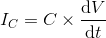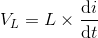# Capacitor and Inductor Charging

## Working principle

In this model, we are showing how the capacitor and inductor behave when connected to an AC source. The table below the waveforms displays the time constants of the inductor (the L/R value) and the capacitor (the RC value). The user may define the time of the step, which switches the source from the capacitor to the inductor.

Notice how the voltage is 90 degrees out of phase with the current (inductor voltage peaks and the capacitor current peaks happen at the maximum slopes of the inductor current and capacitor voltage, respectively). You may relate this to the below equations.

### Useful formulas### Experiment

• Change the parameters of the circuit and verify that the current lags the voltage in an inductive circuit and vice-versa in a capacitive one.